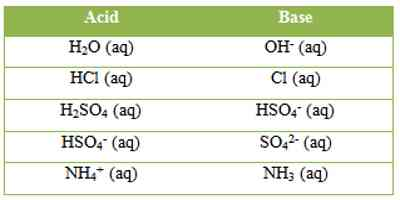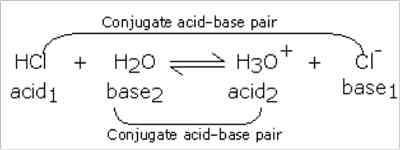# Acids and Bases: Bronsted-Lowry Concept

Acids and Bases: Bronsted-Lowry Concept

In 1923, J. N. Bronsted and J. M. Bjerrum in Denmark and T. M. Lowry in England independently proposed a theory known as ‘the proton theory of acids and bases’. According to this theory-

An acid is a substance – a molecule or an ion – which can donate a proton.

A base is a substance – a molecule or an ion – which can accept a proton.

A proton is a hydrogen atom which has lost its electron. An acid ‘A’ ionizes as-

A (aq) ↔ H+ (aq) + B (aq) … … … (1)

‘B’ is said to be the conjugate base of the acid, while ‘A’ is the conjugate acid of the base ‘B’. A and B are termed conjugate acid-base pair. The conjugate acid and base differ only by a proton. Examples of a few conjugate acid-base pairs are:It may be seen that according to this concept a base or an acid may be a neutral molecule or an ion. Hydrogen chloride acts as an acid in solution because it can donate a proton. If the solvent is water H+ ion forms the hydronium ion, H3O+. Water here acts as a proton acceptor, a base. This can be represented as-..… …. (2)

Ammonia in water acts as a base because it can accept a proton from water. As a result an OH ion is produced,… … … (3)

Water acts as an acid in this reaction. Following the definition it may be said that in equation (2) Cl ion is the conjugate base of the acid HCl, while water is the conjugate base of the acid H3O+. In equation (3) NH3 is the conjugate base of the acid NH4+, while OH is the conjugate base of the acid H2O. It can be clearly seen that conjugate acid-base pairs differ only by a single proton. In general, we can write,

A1 (Acid1) + B2 (Base2) ↔ B1 (Base1) + A2 (Acid2)

Note that depending on the relative proton donating and proton accepting ability the same compound, such as water in the two examples given above, can act either as acid or as a base.

The Bronsted – Lowry concept may also be applied to reaction between HCl and NH3, both dissolved in benzene. In benzene none of these two compounds are ionized. They react, however, to form NH4Cl.